## 2011年3月8日 星期二

### [ Data Structures with Java ] Section 16.1 : Tree Structures

Preface :
A tree is a hierarchical structure that places elements in nodes along branches that originate from a root. Nodes in a tree are subdivided into levels in which the topmost level holds the root node. Any node in a tree can have multiple successors at the next level. Hence, a tree is a nonlinear structure. Operating systems use a general tree to maintain file structures. A node on the tree is a directory. The topmost level is the root directory, and all subsequent levels are subdirectories. Each directory in the tree is recognized by its path name, which is a listing of successive directory names on a path from the root to the node (Below figure a).
Most applications involves a restricted category of trees, called binary trees, in which each node has at mos two successors. For instance, a compiler builds binary trees while parsing expressions in a program's source code. The resulting trees provide information for the portion of the compiler that must interpret the syntax. To illustrate this fact, consider the following arithmetic expression :
a * b + (c - d)/e

The compiler parses the expression and creates an expression tree, in which the variables or constants (a,b,c,d and e) occupy nodes at the end of a path (leaf nodes). The nonleaf nodes contain an operator. In this case, each operator (*, +, -, /) is a binary operator requiring two operands (Below figure b). Before we develop other examples of trees, let use step back and define some terminology that will allow us to describe the features of trees. We will use the terminology to give a precise definition of a binary tree :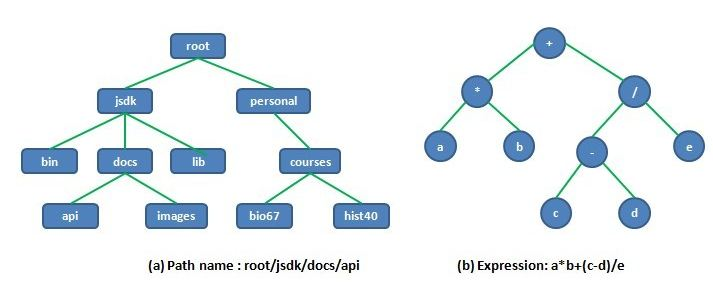Tree Terminology :
A tree structure is characterized as a collection of nodes that originate from a unique starting node called the root. Each node consists of a value and a set of zero or more links to successor nodes. Using analogies from a family tree, we associate the terms parent and child to describe the relationship between a node and any of its successor nodes. In below figure, node A is the root. It is the parent of child nodes B,C and D. The children are siblings. From the perspective of a child, each nonroot node has a unique parent. We classify a node in a tree based on the number of its children. A leaf node, such as E, G, H, I and J is a node without any children. An interior node (nonleaf node) has at least one child. Such as node is sometimes called a internal node :Each node in a tree is the root of a subtree, which consists of the node and all of its descendants. In upper figure, node F is the root of the subtree containing nodes F, I and J while G is a one node subtree. Using the family-tree analogy, a subtree describes a node and all of its descendants. The definition of a subtree permits us to say that node A is a root of a subtree that happens to be the tree itself.
A path between a parent node P and any node N in its subtree is a sequence of nodes. In below figure, the path from root A to node F is the sequence A > C > F with length 2 :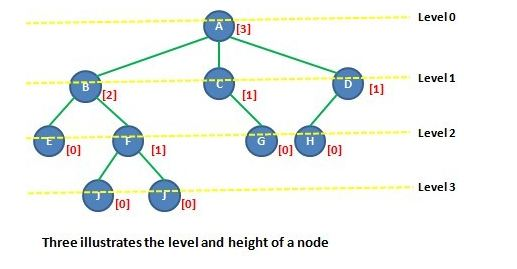In a tree, there is a unique path from the root to any node. The length of the path describes a concept called the level of a node. The level of the root node is 0. Each child of the root is level-1 node, the next generation is level-2 nodes, and so forth. In upper figure, F is a level-2 node. Nodes G and H are level-3 nodes with the longest paths from the root.
The level of a node measures its distance from the root. A related concept is the height of a node. Viewing the node as a root of a subtree, the height is the length of the longest path from the node to a leaf node in the subtree. Clearly, a leaf node has height 0. The heigh of the tree node is the longest path in the tree; that is, the maximum level of the tree. The height of the root is referred to as the height of the tree. For the tree in upper figure, we display the height in brackets adjacent to each node. The tree has height 3.

Binary Trees :
Although general trees have some important applications, we focus on a restricted category of trees, called binary trees, in which each parent has no more than two children. A binary tree has a uniform structure that allows us to give a simple description of its node structure and to develop a variety of tree-handling algorithms. The limit on the number of possible children for a node is not a series restriction because most general trees have an equivalent binary tree representation.
Each node in a binary tree has two links that can reference its children. We distinguish the links by the labels left and right. The left link connects the node to its left childand the right link connects to its right child. Each child is the root of a subtree, so we can also view the left link of each node as a reference to its left subtree and the right link as a reference to its right subtree. In below figure, T is the root of the binary tree with left child L1 and right child R1. The children are roots of the subtrees TL and TR respectively :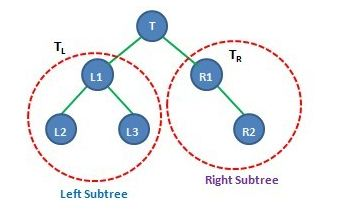Height of a Binary Tree :
The height of a tree is the length of the longest path from the root. In a binary tree, we can use its recursive structure and the concept of tree height to compute the height of a node. If the tree is empty, its height is -1 (stopping condition). The recursive condition applies to a nonempty tree. In this case, the height is defined in terms of the root and its subtrees. The height is one more than the maximum of its subtrees. View a node N as the root of a subtree TN; then :Any leaf node has height 0 using the recursive condition. Each of its subtrees is empty with height -1 and so the leaf node has height 0 = 1 + max(-1,-1). In below figure, the tree has the height 3, which is one more than the height of its left subtree, namely 2. The height of each node is displayed in brackets :The tree in below figure is noteworthy. It has a single leaf node, and all interior nodes have exactly one child. Put another way, each level in the tree contains exactly one node. We use the term degenerate tree to describe such a tree and that it is equivalent to a singly linked list.

Density of a Binary Tree :
In a binary tree, the number of nodes at each level falls within a range of values. At level 0, there is one node, the root; at level 1, there can be 1 or 2 nodes. As we process further, the number of nodes at level 2 is in the range 1 to 4. You see the pattern. Each successive level in the tree can have potentially twice as many nodes as the previous level. At any level k, the number of nodes in the range from 1 to 2^k. The number of nodes per level contributes to the density of the tree. Intuitively, density is a measure of the size of a tree (number of nodes) relative to the height of tree.
Threes with a higher density are important as data structure because they can "pack" more nodes near the root. A potentially large number of nodes reside on relatively short paths from the root. Let us make this idea more precise. Degenerate trees are one extreme measure of density. At the other extreme, a complete binary tree of hight h is a tree in which each level from 0 to h-1 has all possible nodes and all leaf nodes at level h are filled from left to right. The largest possible complete binary tree with hight h is one that contains 2^h nodes at level h. Below figure show a complete binary tree :Evaluating Tree Density :
Complete binary trees are ideal storage structure because of their ability to pack a large number of nodes near the root. A little mathematical analysis determines the minimum hight of a complete tree that hods n elements. Through the first n-1 levels, the total number of nodes is :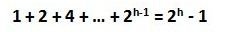At depth h, the number of additional nodes ranges from a minimum of 1 to a maximum of 2^h (full tree). Hence, the number of nodes n in a complete binary tree of height h ranges between :After applying the logarithm base 2 to all terms in the inequality, we have :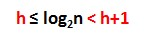This shows that log2n lies between the integer value h and h+1 but cannot equal h+1. Since h must be an integer, the height of the tree is int (log2n). The table below illustrates the calculation for three values of n. Appreciate the fact that for a complete tree with one million nodes, the longest path to any node is at most 19 :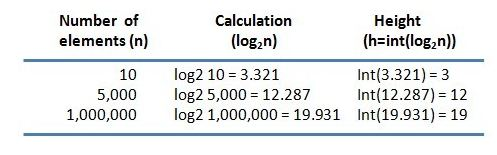### [NodeJS 文章收集] NodeJS accessing file with relative path

Source From  Here Question It seemed like a straight forward problem. But I am not able to crack this. Within  helper1.js  I would like to a...